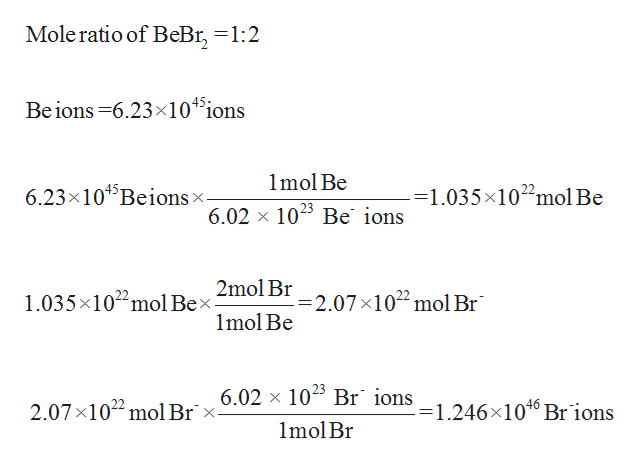The following two elements react to form an ionic compound:1) Be (Z = 4)2) Br (Z = 35)If the compound that is formed by the reaction of these two elements contains 6.23 x 1045 Be ions, calculate how many Br ions the compound contains?Number of Br ions:

Question

The following two elements react to form an ionic compound:
1) Be (Z = 4)
2) Br (Z = 35)

If the compound that is formed by the reaction of these two elements contains 6.23 x 1045 Be ions, calculate how many Br ions the compound contains?

Number of Br ions:

Step 1

Given:

Beryllium ion = 6.23*1045ions

The number of bromide ions pr...help_outlineImage TranscriptioncloseMole ratio of BeBr, =1:2 45 Beions 6.23x10 ions 1mol Be 6.23x104 Beions x. -=1.035x1022mol Be 6.02 x 1025 Be ions 2mol Br 1.035x102 mol Bex -2.07 x102 mol Br 1mol Be 6.02 x 10 Br 1ons_1 246x1040 Br ions 2.07x1022 mol Br x. 1mol Br fullscreen

Want to see the full answer?

See Solution

Want to see this answer and more?

Our solutions are written by experts, many with advanced degrees, and available 24/7

See Solution
Tagged in

Chemistry